## Circular Motion

Linear speed is another way of saying just regular old speed.
Now that we’ve invented the wheel we have the speed at which something rotates: rotational speed or angular speed.

### Which is faster: A or B?

It depends!
The outside of a rotating disc has a greater speed than the inside. AKA tangential speed. So B has a greater linear speed.
At the same time both points complete one trip around the circle in the same time. So they have the same angular or rotational speed.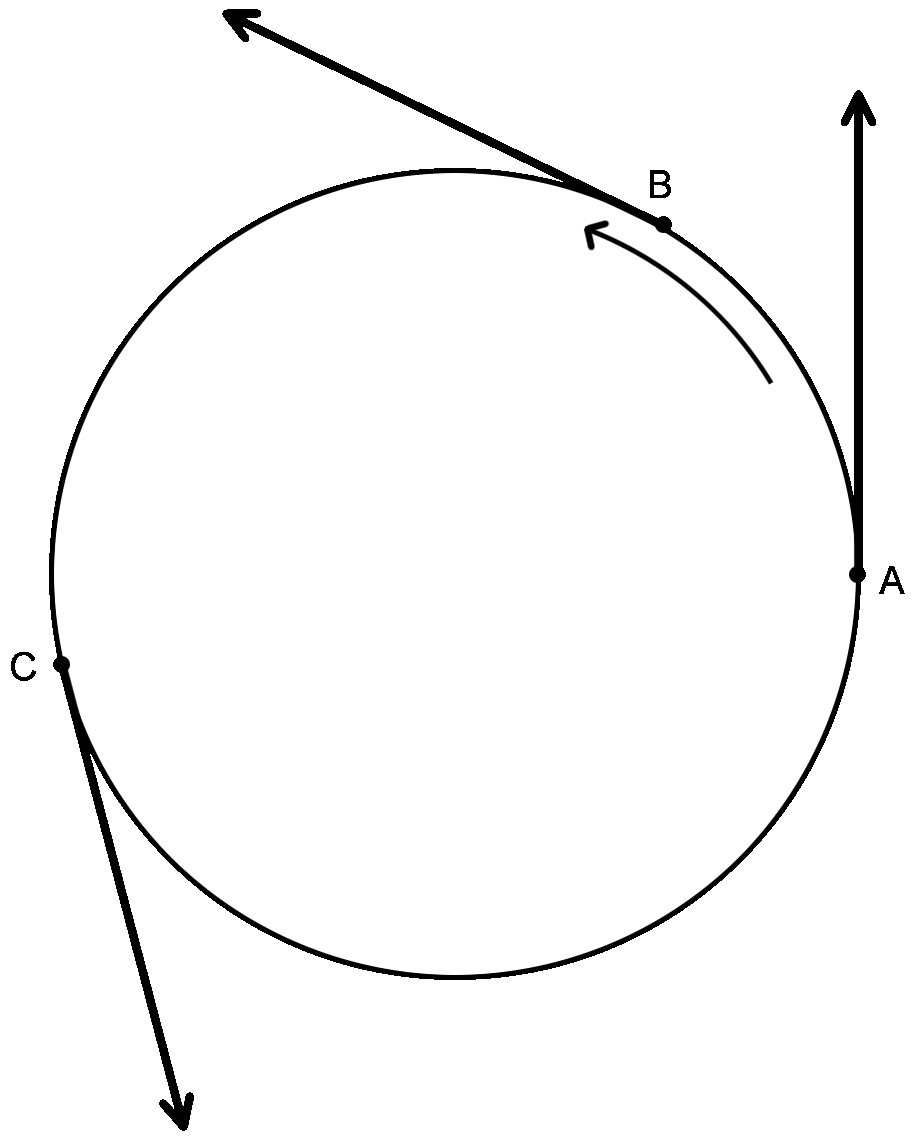In the drawing above, the three dots A, B, C would follow the tangents drawn if they each dislodged from the spinning disc. They DO NOT fly outward in a curving path as many people believe.

It is called tangential speed because if the rotating object were to suddenly dislodge itself from the rotating disc, it would continue going in a straight line from that point at the same speed at which it was currently going. This line would be a tangent to the circle. (Objects in motion stay in motion in a straight line at the same speed unless there is an outside force.)

Sometimes it is called "instantaneous tangential velocity" or just "tangential speed". It is called instantaneous because from moment to moment, the velocity is constantly changing (it direction).

Rotational speed or angular speed is measured in rotations per minute (second etc) otherwise known as RPM's.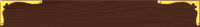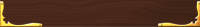## WorksheetsHere's a cool tangent (get it? "tangent" Get it?): A retronym (retro = reverse, nym = name or word) is a word that has to be renamed because something new came along. Confused? Check this out: a hundred years ago if you went into a music store and asked for a guitar. The music dude, let’s call him Sam Ash, reaches up on the shelf and hands you a guitar. Fast forward to today. Same story. But now Sam Ash III asks you “what kind?” Now you have choices: an acoustic guitar or an electric one. What used to be called just plain old “guitar” now has a new name “acoustic guitar” because something new came along and we needed to distinguish which one we wanted. “Liner speed” is kind of like a retronym. We used to call it “speed” but now that we are learning about angular speed we have to distinguish: linear or angular speed.
 Uniform circular motion has the same speed but not velocity. Why? Speed just tells you what your speedometer would read. Velocity is a combination of speed AND direction. In the case of circular motion, this difference becomes important. While traveling in a circle, you are constantly changing your direction. A change in direction changes your velocity and by definition requires an acceleration. Some circular anatomy: Period: The amount of time it takes to make one rotation. The symbol for period is T (how much Time it takes for one cycle). The "T" is capital and often looks rather angry. Frequency is how often it circulates per second (minute…) The symbol for frequency is f. It's a cool graceful italic/script "f". The units for frequency is cycles per second or 1/sec also called Hertz (Hz): “The drill is circulating at 60 cycles per second.” “The drill is circulating at 60Hz.” “The drill has a frequency of 60 cycles per second.” “The drill has a frequency of 60Hz.” -all mean the same thing. The unit 1/sec is a rather strange unit (it’s up there with s2. Really? How can you square a second?) It makes it a little easier to wrap your head around it if you read it as “per second” instead of “1 over seconds” As the time for a cycle increases, the frequency will decrease. If you take a longer time for each cycle, you will get less cycles. As time gets smaller, frequency gets bigger. Frequency and Period are inverses of each other. T = 1/f and f = 1/T Circular velocity = 2 πr/T (v = d/t so in a circle, the distance you travel is 2 πr and divide it by the time T)
 When moving in a circle, velocity is at right angles to the radius or at the tangent to the circle. The direction of velocity is always changing. Changing velocity takes a force. If you suddenly remove the force, the object continues in a straight line - it no longer is pulled into a circle. When driving in a car making a turn you get thrown to the outside because you want to keep moving in a straight line but the door gets in your way.  In order for velocity to constantly change there must be an acceleration.   The acceleration is at right angles to the velocity. If acceleration had any components in the direction of movement, there would be a slowdown or speed up.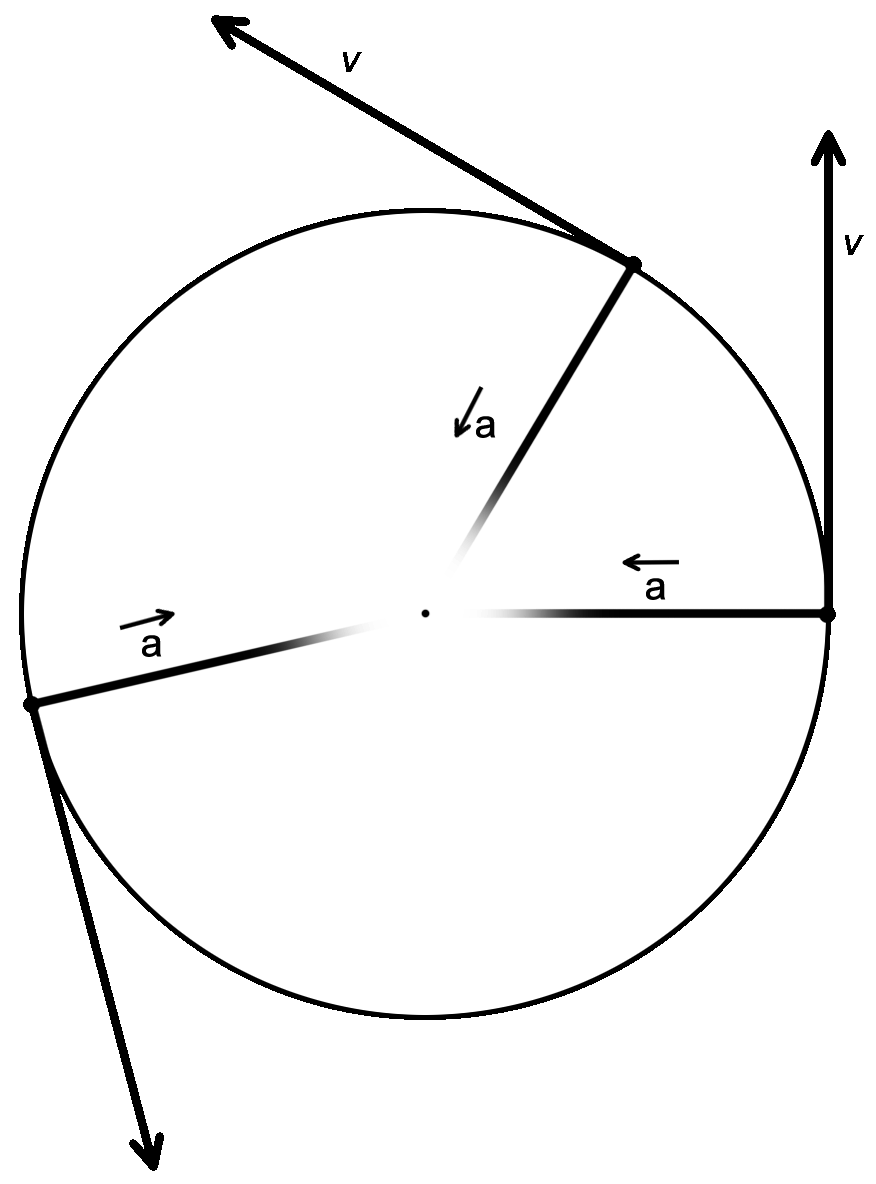## Centripetal vs Centrifugal Forces

Centripetal force is the inward pull that keeps an object moving in a circle when it would otherwise keep going in a straight line.

Even though it is much more famous* than its smarter, better-looking cousin, centrifugal force is actually not a force at all! Objects in motion will stay in motion- a straight line, as long as there are no unbalanced forces working on it. The same is true here. Swing a bucket of water in a circle. The water stays in the bucket. Is it that the water is being thrown outward and the pail stops it?

Sort of kinda yes and no but mostly no.

(*It is made famous by the centrifuge- a device that “spins things to the outside.”)

The water wants to keep going in a straight line tangent to the circle. And it will do so except that the bucket gets in its way because the bucket is being pulled inward into a curve.

The most common example usually used to illustrate this principal is riding in a car on a turn. You are in the passenger seat and the driver makes a sudden left turn. It feels like you are being thrown to the outside of the car. What is actually happening is that your inertia is attempting to continue in a straight line but the car door turns in front of you and pushes you in towards the center of the curve.

 Here’s a way to visualize this fight between inertia, centripetal force, centrifugal force, and centripetal acceleration: Your inertia wants you to continue in a straight line but the red force arrow yanks you back on course with the circular track. From that point you continue on your straight line tangent again. Only to be yanked back. But this time the course correction didn’t take as long and the yank isn’t as hard nor is the course correction as drastic. Each correction comes quicker and quicker. This makes each correction smaller and less forceful. Finally, you constantly are making tiny corrections with a very small force. Also notice that at first, the major correctional force yanked back at this rather severe acute angle (actually it is a very open obtuse angle where you are almost turned around 180º). But as the corrections got smaller and closer to each other the angle got closer to a right angle.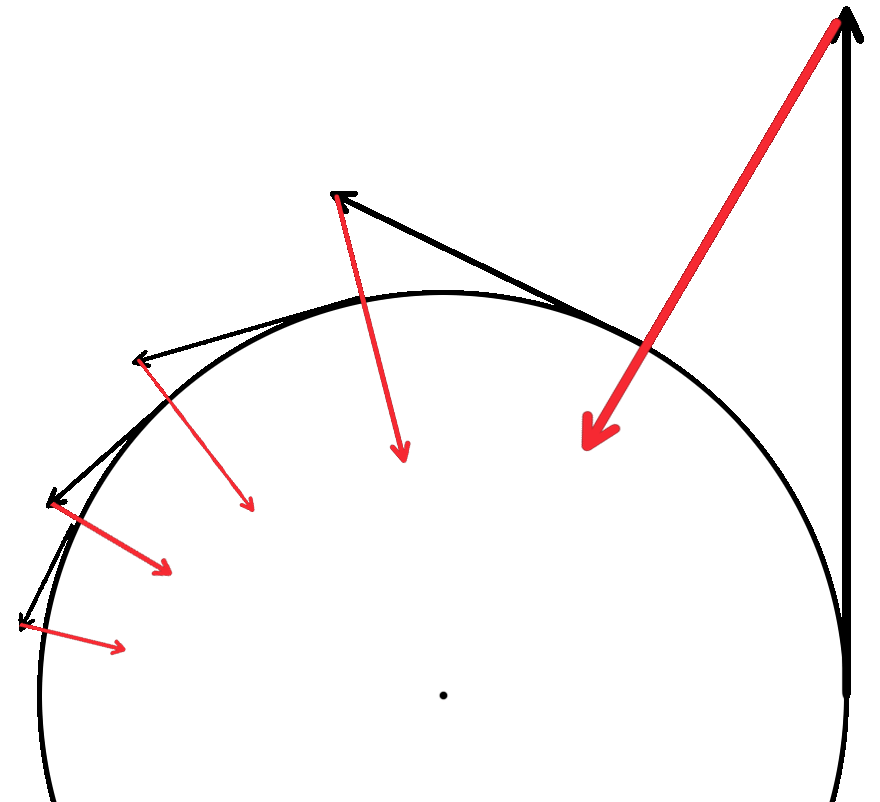## ac = v2/r

ac is centripetal acceleration
v is the tangential velocity (speed)

a and v are directly proportional. Acceleration towards the center will need to increase as speed increases.

a and r are inverses. As the radius gets smaller acceleration gets higher.

I like to think about it in terms of the planets. Mercury is closest to the sun. It feels the greatest acceleration of gravity from the sun. Its velocity is big and its radius from the sun is small

## Fc = mac

This is the same as our old friend except substituted with the circular terms.

## Sample circular motion problem #1: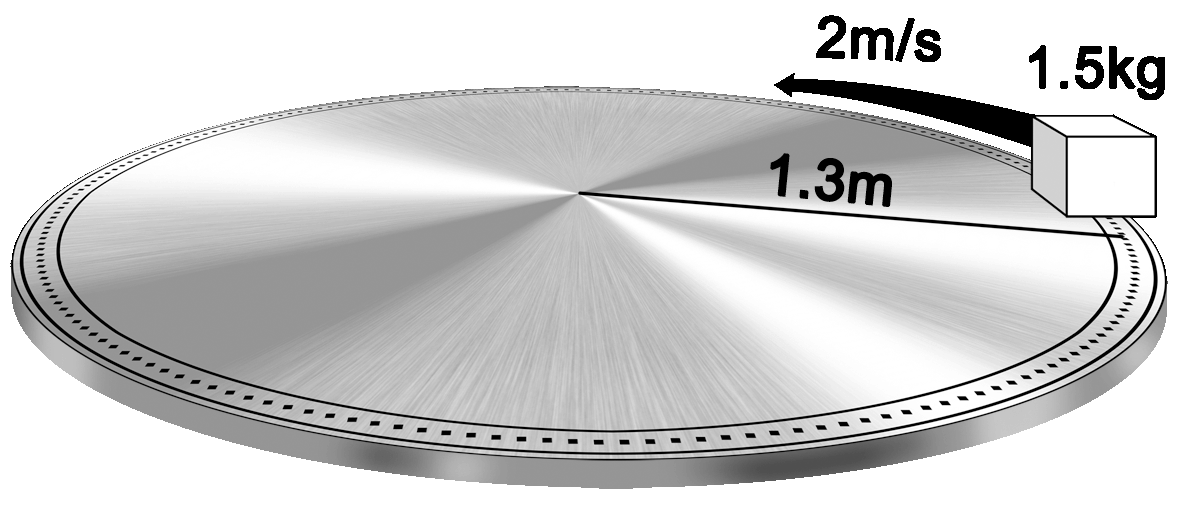A cart is going around a track with a radius of 1.3 meters. The cart has a mass of 1.5kg and is moving at 2m/s.

What is the centripetal acceleration (ac)?

What is the Fentripetal force (Fc)?

ac = v2/r

(2m/s)(2m/s)/(1.3m)

3.1m/s2

Fc = mac

= (1.5kg)(3.1m/s2)

= 4.6kgm/s2 (4.6N)

## Sample circular motion problem #2:

A 1000kg car going around a turn with a speed of 20m/s experiences a centripetal acceleration of 40m/s2.

What is the radius of the turn?

What is the centripetal acceleration?

ac = v2/r -> r = v2/a

(20m/s)(20m/s)/(40m/s2)

10m

Fc = mac

= (1000kg)(40m/s2)

= 40,000N (or 4 x 104N)

## Sample circular motion problem #3:

An astronaut with a mass of 75kg is on a space station that it 100m across. The station (and astronaut) rotates with a linear speed of 31.32m/s.

Find the ac and Fc

ac = v2/r

(31.32m/s)(31.32m/s)/(100m)

ac = 9.81m/s2

Fc = mac

= (75kg)(9.81m/s2)

= 735.8N

The significance of this problem is that the space station rotates in such a way that the centripetal acceleration is 9.81m/s2 which give the astronaut a centripetal force of 735.8N. These are the same values that he would have on Earth for the ag and mg (weight).

This essentially creates artificial gravity and will allow the astronaut to walk on the inner side of the outside of the ring.

Below is an artist’s concept of a ringed ship with artificial gravity from Battle Star Galactica.
(http://drexfiles.wordpress.com/category/bsg/)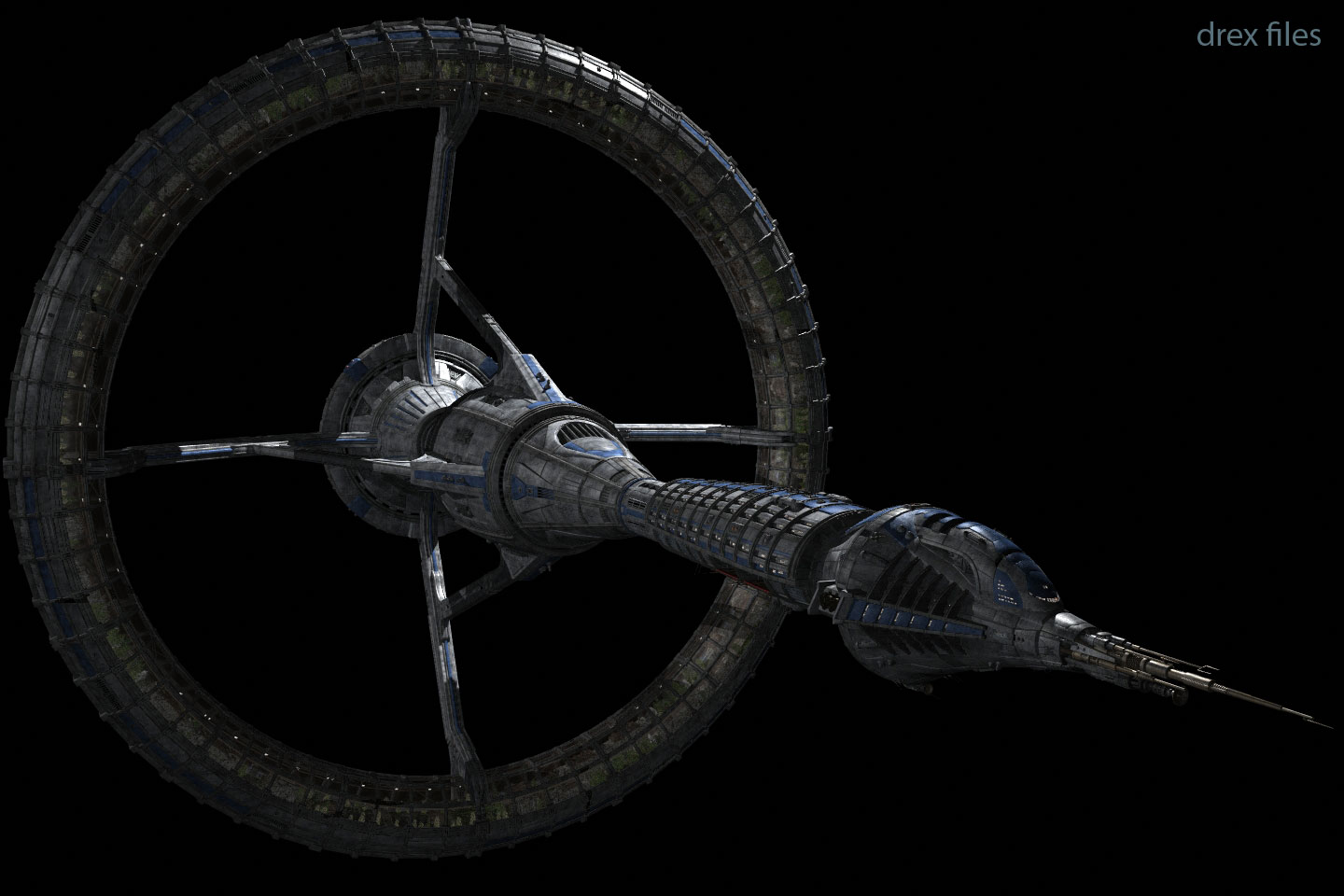## Circular Motion Lab

Circular Motion Lab

Objective: To calculate the mass of an object by measuring its circular velocity, acceleration and forces.

### Materials:

• Circular motion apparatus with attached Newton meter
• Meter stick
• Stop watch
• Electronic balance

### Procedure:

1. Measure the mass of the weight on the end of the string.
2. Arbitrarily select a force on the Newton meter. Ex: 2N
3. Swing the mass above your head in a horizontal circle in such a way that the newton meter consistently reads 2N.Be sure to move the center bar as little as possible.
4. While swinging the mass, a partner will measure the time for 10 complete swings.
5. Once finished with the swinging, measure the length of the radius of the string- be sure to pull the string out until the Newton meter reads 2N.
6. Repeat the measurements for a total of 5 trials with different forces.

Put the data into a chart:

 mass(kg) =

 Trial# F (N) r (m) T for 1 spin (sec) v (2 πr/T) Ac (v2/r) 0 0 0 0 0 0 1 2 3 4 5

### Calculations:

Calculate v using v = 2πr/T and insert into the chart.
Calculate a using Fc = mac and insert into the chart.

To find relationship between ac and v graph:

• ac vs v
• ac vs v2

To calculate mass:

Graph Fc vs ac

Mass will be the slope of the line

Check you accuracy by calculating % error- using the measured mass from the balance and the calculated mass from the graph.

% error = difference from accepted value / accepted value x100

Where:

• "difference from accepted value" is the difference between the value you calculate and the value measured on the electronic balance.
• "accepted value" is the value you measured on the electronic balance.

## Worksheets:Medina On-Line
Since 1994
Email: mrsciguy@optonline.net